Updating search results...

# 38 Results

View
Selected filters:
• CCSS.Math.Content.HSA-SSE.A.1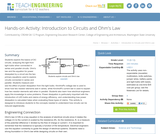Educational Use
Rating
4.0 stars

Students explore the basics of DC circuits, analyzing the light from light bulbs when connected in series and parallel circuits. Ohm's law and the equation for power dissipated by a circuit are the two primary equations used to explore circuits connected in series and parallel. Students measure and see the effect of power dissipation from the light bulbs. Kirchhoff's voltage law is used to show how two resistor elements add in series, while Kirchhoff's current law is used to explain how two resistor elements add when in parallel. Students also learn how electrical engineers apply this knowledge to solve problems. Power dissipation is particularly important with the introduction of LED bulbs and claims of energy efficiency, and understanding how power dissipation is calculated helps when evaluating these types of claims. This activity is designed to introduce students to the concepts needed to understand how circuits can be reduced algebraically.

Subject:
Engineering
Electronic Technology
Material Type:
Activity/Lab
Provider:
TeachEngineering
Provider Set:
TeachEngineering
Author:
Erik Wemlinger
09/18/2014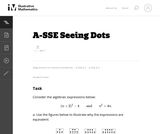Unrestricted Use
CC BY
Rating
4.0 stars

The purpose of this task is to identify the structure in the two algebraic expressions by interpreting them in terms of a geometric context. Students will have likely seen this type of process before, so the principal source of challenge in this task is to encourage a multitude and variety of approaches, both in terms of the geometric argument and in terms of the algebraic manipulation.

Subject:
Mathematics
Algebra
Material Type:
Activity/Lab
Provider:
Illustrative Mathematics
Provider Set:
Illustrative Mathematics
Author:
Illustrative Mathematics
05/17/2013Conditional Remix & Share Permitted
CC BY-NC
Rating
0.0 stars

Modeling Our World with Mathematics Unit 4: Finances for Life Topic 3 - Business

Subject:
Mathematics
Material Type:
Module
Author:
Hannah Hynes-Petty
Washington OSPI Mathematics Department
Washington OSPI OER Project
11/20/2020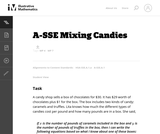Unrestricted Use
CC BY
Rating
0.0 stars

This task assumes students are familiar with mixing problems. This approach brings out different issues than simply asking students to solve a mixing problem, which they can often set up using patterns rather than thinking about the meaning of each part of the equations.

Subject:
Mathematics
Algebra
Material Type:
Activity/Lab
Provider:
Illustrative Mathematics
Provider Set:
Illustrative Mathematics
Author:
Illustrative Mathematics
05/01/2012Unrestricted Use
CC BY
Rating
0.0 stars

Subject:
Mathematics
Algebra
Material Type:
Activity/Lab
Provider:
Illustrative Mathematics
Provider Set:
Illustrative Mathematics
Author:
Illustrative Mathematics
05/01/2012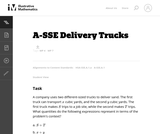Unrestricted Use
CC BY
Rating
0.0 stars

The primary purpose of this task is to illustrate certain aspects of the mathematics described in the A.SSE.1. The task has students look for structure in algebraic expressions related to a context, and asks them to relate that structure to the context. In particular, it is worth emphasizing that the task requires no algebraic manipulation from the students.

Subject:
Mathematics
Algebra
Material Type:
Activity/Lab
Provider:
Illustrative Mathematics
Provider Set:
Illustrative Mathematics
Author:
Illustrative Mathematics
05/01/2012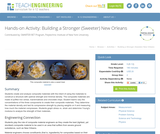Educational Use
Rating
0.0 stars

Students create and analyze composite materials with the intent of using the materials to construct a structure with optimal strength and minimal density. The composite materials are made of puffed rice cereal, marshmallows and chocolate chips. Student teams vary the concentrations of the three components to create their composite materials. They determine the material density and test its compressive strength by placing weights on it and measuring how much the material compresses. Students graph stress vs. strain and determine Young's modulus to analyze the strength of their materials.

Subject:
Architecture and Design
Engineering
Material Type:
Activity/Lab
Provider:
TeachEngineering
Provider Set:
TeachEngineering
Author:
Charisse Nelson
Sarah Wigodsky
10/14/2015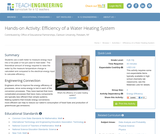Educational Use
Rating
0.0 stars

Students use a watt meter to measure energy input into a hot plate or hot pot used to heat water. The theoretical amount of energy required to raise the water by the measure temperature change is calculated and compared to the electrical energy input to calculate efficiency.

Subject:
Engineering
Physics
Material Type:
Activity/Lab
Provider:
TeachEngineering
Provider Set:
TeachEngineering
Author:
Jan DeWaters
Susan Powers
09/18/2014Conditional Remix & Share Permitted
CC BY-NC
Rating
0.0 stars

In this unit of study students learn that in the horizontal direction a projectile moves at a constant speed with nothing to cause acceleration. In the vertical direction a projectile accelerates due to the earth’s gravitational field. And combining these two type of motions together you can determine the parabolic arch of a projectile. This unit integrates nine STEM attributes and was developed as part of the South Metro-Salem STEM Partnership's Teacher Leadership Team. Any instructional materials are included within this unit of study.

Subject:
Physics
Material Type:
Unit of Study
Provider:
South Metro-Salem STEM Partnership
Author:
Sarah Price
04/29/2015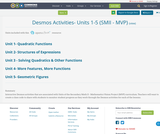Conditional Remix & Share Permitted
CC BY-SA
Rating
0.0 stars

Interactive Desmos activities that are associated with Units of the Secondary Math II - Mathematics Vision Project (MVP) curriculum.
Teachers will want to create a class code to share with students to monitor student progress as they work through the Desmos activities for each of the lessons.

Subject:
Mathematics
Material Type:
Interactive
Author:
Mindy Branson
10/24/2019Conditional Remix & Share Permitted
CC BY-NC
Rating
0.0 stars

Modeling Our World with Mathematics Unit 1: Health & Fitness Topic 2 - Sports & Fitness

Subject:
Mathematics
Material Type:
Module
Author:
Hannah Hynes-Petty
Washington OSPI Mathematics Department
Washington OSPI OER Project
09/29/2020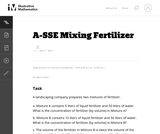Unrestricted Use
CC BY
Rating
0.0 stars

The problem deals with a rational expression which is built up from operations arising naturally in a context: adding the volumes of the fertilizer and the water, and dividing the volume of the fertilizer by the resulting sum. Thus it encourages students to see the expression as having meaning in terms of numbers and operations, rather than as an abstract arrangement of symbols.

Subject:
Mathematics
Algebra
Material Type:
Activity/Lab
Provider:
Illustrative Mathematics
Provider Set:
Illustrative Mathematics
Author:
Illustrative Mathematics
05/01/2012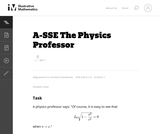Unrestricted Use
CC BY
Rating
0.0 stars

Subject:
Mathematics
Algebra
Physics
Material Type:
Activity/Lab
Provider:
Illustrative Mathematics
Provider Set:
Illustrative Mathematics
Author:
Illustrative Mathematics
05/01/2012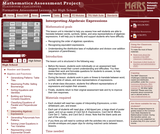Only Sharing Permitted
CC BY-NC-ND
Rating
0.0 stars

This lesson unit is intended to help teachers assess how well students are able to translate between words, symbols, tables, and area representations of algebraic expressions. It will help teachers to identify and support students who have difficulty in: recognizing the order of algebraic operations; recognizing equivalent expressions; and understanding the distributive laws of multiplication and division over addition (expansion of parentheses).

Subject:
Algebra
Material Type:
Assessment
Lesson Plan
Provider:
Shell Center for Mathematical Education
Provider Set:
Mathematics Assessment Project (MAP)
04/26/2013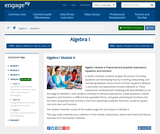Conditional Remix & Share Permitted
CC BY-NC-SA
Rating
0.0 stars

In earlier modules, students analyze the process of solving equations and developing fluency in writing, interpreting, and translating between various forms of linear equations (Module 1) and linear and exponential functions (Module 3). These experiences combined with modeling with data (Module 2), set the stage for Module 4. Here students continue to interpret expressions, create equations, rewrite equations and functions in different but equivalent forms, and graph and interpret functions, but this time using polynomial functions, and more specifically quadratic functions, as well as square root and cube root functions.

Subject:
Algebra
Material Type:
Module
Provider:
New York State Education Department
Provider Set:
EngageNY
09/17/2013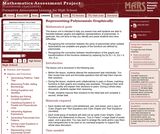Only Sharing Permitted
CC BY-NC-ND
Rating
0.0 stars

This lesson unit is intended to help teachers assess how well students are able to translate between graphs and algebraic representations of polynomials. In particular, this unit aims to help you identify and assist students who have difficulties in: recognizing the connection between the zeros of polynomials when suitable factorizations are available, and graphs of the functions defined by polynomials; and recognizing the connection between transformations of the graphs and transformations of the functions obtained by replacing f(x) by f(x + k), f(x) + k, -f(x), f(-x).

Subject:
Mathematics
Material Type:
Assessment
Lesson Plan
Provider:
Shell Center for Mathematical Education
Provider Set:
Mathematics Assessment Project (MAP)
04/26/2013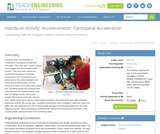Educational Use
Rating
0.0 stars

Students work as physicists to understand centripetal acceleration concepts. They also learn about a good robot design and the accelerometer sensor. They also learn about the relationship between centripetal acceleration and centripetal force governed by the radius between the motor and accelerometer and the amount of mass at the end of the robot's arm. Students graph and analyze data collected from an accelerometer, and learn to design robots with proper weight distribution across the robot for their robotic arms. Upon using a data logging program, they view their own data collected during the activity. By activity end , students understand how a change in radius or mass can affect the data obtained from the accelerometer through the plots generated from the data logging program. More specifically, students learn about the accuracy and precision of the accelerometer measurements from numerous trials.

Subject:
Engineering
Physics
Material Type:
Activity/Lab
Provider:
TeachEngineering
Provider Set:
TeachEngineering
Author:
Carlo Yuvienco
Jennifer S. Haghpanah
09/18/2014Conditional Remix & Share Permitted
CC BY-NC
Rating
0.0 stars

Modeling Our World with Mathematics Unit 4: Finances for Life Topic 1 - Introduction to Finance

Subject:
Mathematics
Material Type:
Module
Author:
Hannah Hynes-Petty
Washington OSPI Mathematics Department
Washington OSPI OER Project
10/12/2020Only Sharing Permitted
CC BY-NC-ND
Rating
0.0 stars

This lesson unit is intended to help teachers assess how well students are able to interpret exponential and linear functions and in particular to identify and help students who have the following difficulties: translating between descriptive, algebraic and tabular data, and graphical representation of the functions; recognizing how, and why, a quantity changes per unit intervale; and to achieve these goals students work on simple and compound interest problems.

Subject:
Algebra
Measurement and Data
Material Type:
Assessment
Lesson Plan
Provider:
Shell Center for Mathematical Education
Provider Set:
Mathematics Assessment Project (MAP)
04/26/2013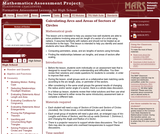Only Sharing Permitted
CC BY-NC-ND
Rating
0.0 stars

This lesson unit is intended to help teachers assess how well students are able to solve problems involving area and arc length of a sector of a circle using radians. It assumes familiarity with radians and should not be treated as an introduction to the topic. This lesson is intended to help teachers identify and assist students who have difficulties in: Computing perimeters, areas, and arc lengths of sectors using formulas and finding the relationships between arc lengths, and areas of sectors after scaling.

Subject:
Geometry
Material Type:
Assessment
Lesson Plan
Provider:
Shell Center for Mathematical Education
Provider Set:
Mathematics Assessment Project (MAP)